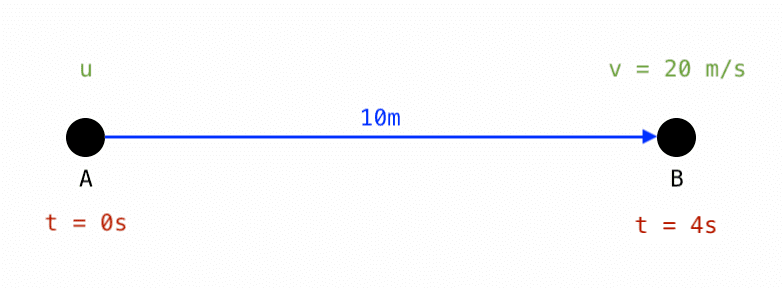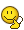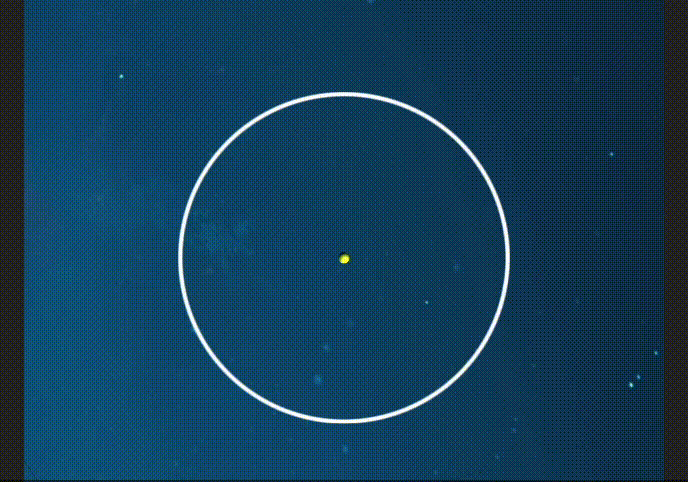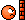# Moving in a straight line with multiple constraints

• Jack7

#### Jack7

Homework Statement
Linear motion challenge
Relevant Equations
SUVAT?
Hi All,

I am trying to solve what I think should be a simple problem, but I must be missing something because I am struggle to solve it. The situation is shown below:So to summarise:
• A body starts at Point A
• It moves in a straight line to Point B, covering a distance of 10m
• The time taken to travel this distance is 4 seconds
• When it reaches Point B, it has a velocity of 20 m/s

I need to write a computer program to animate this motion, but I am stuck. How do I find the rate of acceleration? The acceleration could be linear or non-linear, and I do not know what the initial velocity (u) is. Ideally I want it to be 0.

So effectively, I am trying to create this motion by imposing the values listed above, because I need the object to follow this very specific profile of motion.

When I try the SUVAT equations, if I use u = 0, I get a linear acceleration of a = 1.25 m/s (a = 2s / t^2). But then when I calculate the velocity at Point B using this value, v = u + at gives me 5 m/s. This is incorrect as I need it to be 20 m/s as shown above. So I am assuming the SUVAT equations do not work in this case? Do I need to use a non-linear method?

Any help would be greatly appreciated! Please explain the steps if possible, rather than advising something broad like drawing a curve and finding the integral. I need some clear steps to follow.

Homework Statement:: Linear motion challenge
Relevant Equations:: SUVAT?

Do I need to use a non-linear method?
Your problem is that if you assume constant acceleration then you already have enough information to determine everything, including the initial velocity (which is not zero).
If you insist on starting from rest then it will be varying acceleration. That opens up a wealth of possibilities. You could have it undergo two different accelerations (one being zero perhaps, or even negative), or make the rate of change of acceleration constant. That last option might involve solving a cubic.

•PeroK
The SUVAT equations does not work because you are assuming u = 0.

Is u = 0 a must? Then you can not use constant acceleration.

Will your simulation take u as an input? We need some more details.

Thanks guys for your quick responses!

Okay so u does not have to equal 0. If I can create a constant acceleration with a higher value for u, then that's fine. So long as it arrives at Point B within the specified time, at the specified final velocity, across the specified distance. How then can I find u?

Last edited:
•malawi_glenn
Thanks guys for your quick responses!

Okay so u does not have to equal 0. If I can create a constant acceleration with a higher value for u, then that's fine. So long as it arrives at Point B within the specified time, across the specified distance. How then can I find u?
Use the SUVAT equations but with u ≠ 0.
Just redo what you did, but solve for u and a in a system of equations.
There is another way, there is a SUVAT equation that connects distance, constant acceleration, start and final velocities, but does not include time. Can you figure out which formula I am referring to and why it is good to use?

I think my problem is that I am not taking the correct approach to solving these equations. If I consider the recommendation above then:

S = 10
U = ?
V = 20
A = ?
T = 4

If I use s = ut + 1/2at^2 then I arrive at:

10 = 4u + 8a. Solving this gives u = 0.5 and a = 1.

But then if I test these values to check V after 4 seconds, I get v = u + at which gives v = 0.5 + (1 * 4) = 4.5.

Obviously this is incorrect as V needs to be 20. It's been a while since I touched any equations so clearly I need to go back to school! Gah!

10 = 4u + 8a. Solving this gives u = 0.5 and a = 1.
You have only one equation there, with two unknowns. For instance u = 0.25 m/s gives a = 9/8 m/s2.
The space of solutions form a straight line.

You need one more equation to uniqely solve for u and a in your problem. Hence, you need to solve a system of two equations, with two unknowns. What other equation do you think we need here?

Did you give some thought on my hint of looking at a different SUVAT equation, that does no involve t?

Last edited:
I think my problem is that I am not taking the correct approach to solving these equations. If I consider the recommendation above then:

S = 10
U = ?
V = 20
A = ?
T = 4
The trick with SUVAT is to identify the variable you do not care about and pick the equation which omits it. Can you find one that omits a?

•Delta2 and malawi_glenn
@malawi_glenn Yes I see what you mean, I am solving for one equation which is not enough. I think you might be referring to v^2 = u^2 + 2as.

Therefore, 400 = u^2 + (2 x 10 x a). So I now have two equations:

10 = 4u + 8a
400 = u^2 + 20a

I'm guessing I need to substitute one equation into the other to solve for either u or a, and then use that value to find the corresponding other. Is that right?

•malawi_glenn
I'm guessing I need to substitute one equation into the other to solve for either u or a, and then use that value to find the corresponding other. Is that right?

Yes, but now that I have had my third cup of coffee, I think you are better of with the SUVAT equations that you started with
##s = ut + \dfrac{at^2}{2}##
##v = u + at##
Insert your values for v, t and s. Solve for u and a.

•SammyS
My gosh, I must be being incredibly dim right now. I cannot seem to solve this basic problem. Following on from the above, we get:

t = 4, v = 20, s = 10

Hence,

10 = 4u + 8a (s = ut + 1/2at^2)
20 = u + 4a (v = u + at)

Re-arranged the top one in order to solve,

2.5 = u + 2a
20 = u + 4a

Combining to solve a,

17.5 = 2a , therefore a = 8.75

Solving for u,

u = 20 - 4(8.75) = -15

So, u = -15, a = 8.75.

If I plug this into s = ut + 1.2at^2, it gives me the correct value of s = 10m
If I plug this into v = u + at, it gives me the correct value of v = 20m/s

BUT, it makes no sense! How can 'u' be negative? I am completely confused.

__

Furthermore, if I try to find what the displacement would be say at t = 2s, if I substitute into s = ut + 1/2at^2 given the u and t above, it gives a distance of -12.5. This is obviously incorrect, because the displacement must be at some point between 0 and 10.

Last edited:
v^2 = u^2 + 2as.

##s = ut + \dfrac{at^2}{2}##
The trouble with both of those is that they involve both unknowns, a and u. Using either will mean you need to use two SUVAT equations and solve as a pair of simultaneous equations.
As I wrote in post #8, the easy way is to find a SUVAT equation with only one unknown. Omitting a, you have ##s=\frac 12(v+u)t##; omitting u, ##s = vt - \dfrac{at^2}{2}##.

How can 'u' be negative?

the displacement must be at some point between 0 and 10
It is surprising but true. For a constant acceleration solution, the particle has to start going away from the target but accelerate towards it. In that way it gets a good run up and can reach the desired velocity at a relatively low acceleration, and hence avoid arriving too soon.

It is surprising but true. For a constant acceleration solution, the particle has to start going away from the target but accelerate towards it. In that way it gets a good run up and can reach the desired velocity at a relatively low acceleration, and hence avoid arriving too soon.

Ohhhhhh! That is incredibly intriguing! I understand what you mean. My assumption that the displacement at t = 2s must be somewhere between 0 and 10m is based on a misconception that it can only move in one direction. There is in fact nothing in my scenario that explicitly constrained the direction.

I suppose I intuitively assumed one direction of travel given the arrow from A to B. So does that mean in order to get from A to B in ONLY that direction in accordance with the specified S,T,V, one must use non-linear acceleration from rest (where rest is at A)?

I think I would prefer this, as I do not want my animation to swing left and then suddenly start accelerating right. I would prefer one direction of motion.

in order to get from A to B in ONLY that direction in accordance with the specified S,T,V, one must use non-linear acceleration from rest (where rest is at A)?
Yes. I mentioned a couple of options in post #2.
A trivial one is to stay still until accelerating at 20m/s2 gets to B at the right time, but that will not look different from the case of accelerating constantly at that rate from the start and arriving too soon.

Yes. I mentioned a couple of options in post #2.
A trivial one is to stay still until accelerating at 20m/s2 gets to B at the right time, but that will not look different from the case of accelerating constantly at that rate from the start and arriving too soon.

Why an acceleration of 20m/s2? How did you arrive at this figure?

Why an acceleration of 20m/s2? How did you arrive at this figure?
it was an example of a time dependent acceleration. Until some time t0 the particle is experience no acceleration. But once t > t0 then it starts to move with acceleration 20 m/s2

it was an example of a time dependent acceleration. Until some time t0 the particle is experience no acceleration. But once t > t0 then it starts to move with acceleration 20 m/s2

Ah I see, I'm not sure I fully understand this but I think I get the gist of it, i.e. breaking the acceleration up into two phases.

I think the previous exercise was very useful to understand why a linear acceleration was not appropriate. But as it stands I am back to square one, now trying to accelerate from rest. I need the particle to move rightwards, covering the specified distance within the specified time, ending with the specified velocity.

Please can you advise how I might go about this? The advice in Post #2 was very broad so I don't know how to make use of it. Do I need to find a t0? Effectively I need to be able to determine the displacement 's' as a function of time 't'. For example, at = 2s, I need to know where between 0 and 10m the particle is. And it must reach B with v = 20m/s. Any practical pointers would be greatly appreciated.

I also really appreciate the two of you helping me get this far, it is a huge help so thank you very much.

Ok, so the particle is not allowed at any point in time move to the left, did I get that right?

•Jack7 and Delta2
Ok, so the particle is not allowed at any point in time move to the left, did I get that right?

That is absolutely correct

•Delta2
That is absolutely correct
You could try different acceleration functions, like exponential function ##a(t) = C\cdot e^{kt}## or power function ##a(t) = k\cdot t^{\alpha -2}##, integrate and solving for the unknown constants using your constraints.
Or, a piece-wise constant acceleration ##a(t) = a_1## for ## 0 \leq t <t_1## and ##a(t) = a_2## for ## t_1 \leq t ##

You can play around with those, by setting u = 0 and see what kind of motions you will end up with

•Jack7
Suppose a low acceleration a1 for time t and a greater acceleration a2 for the rest. That's three unknowns, which is one too many for the given constraints, so you can in principle pick a value for anyone and solve for the other two. But for some choices you will still end up getting illegal moves, so you will have to play about with your choice until it gives a valid solution.

Basically, for a continuous solution you want ## v## to be in a single direction. If the position coordinate is ##x##, then you want something to the effect of:

$$v = \frac{dx}{dt} = c e^{kt}$$

which @malawi_glenn has already pointed out.

•Jack7, Delta2 and malawi_glenn
Sorry guys but my math skills are very rusty at this moment in time. I see a differential equation but how to practically make use of that eludes me entirely. What is "C" and "k" shown above?

If someone could outline some clear, practical steps to follow it would be a big help. For example how do I find C, or k? How does this help me find displacement at a given time? Sorry if these are obvious questions.

What is "C" and "k" shown above?
Those are constants which you need to find given your constraints.

For instance of you choose to play with ##a(t) = C\cdot e^{kt}## and ##v(0) = u = 0## then you need to integrate this acceleration twice. The first time you integrate it you get the velocity function, and the second time you get the position function. Your constraints are v(4) = 20 and s(4) = 10 using s(0) = 0.

Okay, I need some time to think about this in more detail, since I haven't done any calculus in quite some timeI'll try to post some results back in a few hours, thanks again to you all for your recommendations!

•erobz
Once you know how it will work for u = 0, you can solve the equations of motion for any (positive) u.

Okay, I need some time to think about this in more detail, since I haven't done any calculus in quite some timeI'll try to post some results back in a few hours, thanks again to you all for your recommendations!
Yeah, it's probably best to try to refamiliarize yourself with the process and post some attempt so people can see where any gaps may be.

EDIT: try to use LaTeX for your solution when replying. It doesn't take very long to learn, and it makes the math easily readable. There is always a link under the reply box for your reference.

LaTeX Guide

Last edited:
••malawi_glenn and Delta2
I need to write a computer program to animate this motion
In some contexts we call these animations easings. You can find out more about easings at https://easings.net/ (no kidding!)

A useful easing when you want to specify slope (velocity) at the beginning and end of the transition is a cubic spline defined by $$x(t) = \alpha t^3 + \beta t^2 + \gamma t$$ (we define ## x(0) = 0 ## so there is no constant coefficent). We can differentiate this to get an equation for ## \dot x(t) ##, and we know that ## x(T) = S = 10m, \dot x(0) = 0 ## and ## \dot x(T) = V = 20 m/s ##. This gives us 3 equations in 3 unknowns which can be solved easily to get ## \alpha ## and ## \beta ## in terms of ## S, V ## and ## T ## (we can quickly see that ## \gamma = 0 ##).

Oh,You may not know that we have other topics that may have been more appropriate for this question that appears to be more about computer programming than physics, particularly https://www.physicsforums.com/forums/engineering-and-comp-sci-homework-help.158/ and https://www.physicsforums.com/forums/programming-and-computer-science.165/, however it's probably best to leave this one here for now.

If you want to talk more about implementing this in a particular language I should start a new topic, either in the homework topic if it is a problem that you need to solve as part of your studies, or the general Programming and Computer Science topic if not.

Last edited:
•Jack7
For instance of you choose to play with ##a(t) = C\cdot e^{kt}## and ##v(0) = u = 0## then you need to integrate this acceleration twice. The first time you integrate it you get the velocity function, and the second time you get the position function. Your constraints are v(4) = 20 and s(4) = 10 using s(0) = 0.
That would be an exponential easing https://easings.net/#easeInExpo. It will work, but because nearly all the motion happens at the end of the period it is usually less satisfactory than cubic. Also it is much easier to start with an equation for position and differentiate rather than one for acceleration and integrate.

•Jack7
Honestly guys I think I've hit a brick wall. I struggle to make sense of the equations above since they are too abstract, it's been too long since I did any proper maths! I have however tried to find a solution based on my understanding (or lack thereof!) of the above recommendations. Here is what I did:
1. Plot a velocity time graph where u = 0, v = 20, t = 4. The shape of this graph is something I arbitrarily set to an exponential shape, given that this is the profile I am looking for. I think you guys were recommending this above too.
2. Use step wise integrals to find the area under the graph, in order to create a corresponding displacement time graph. Remember that I said I need displacement as a function of time. (I have a function that can do this accurately for as many intervals as needed).
3. Now Step 2 doesn't take into account my distance of s = 10m since it only looks at the velocity time graph, so the y-axis of my displacement time graph is not correctly scaled. Therefore I scale it to match my displacement profile of s = 10.
4. I move the particle using this displacement time graph.

The result of this process is below:The motion discussed in this thread is the motion from off-screen right up until it hits the circle directly below the yellow point. After that the particle follows a circular motion with an angular velocity matched to the linear velocity. So if it works, we should see a nice smooth transition.

You can see however that it has not worked. I get an acceleration but it is too rapid, which makes the particle suddenly slow down after the linear motion has occurred. I assume that I have made a mistake in Steps 2-3 because I am effectively experimenting here without actually knowing what I am doing.If anyone could please outline where I've gone wrong, I'd really appreciate it.

If anyone could please outline where I've gone wrong,
Very hard to tell if you do not show any equations.

So your ultimate goal is for that square to start at a distance 10 m from the lowest point of a circle, accelerate towards it in 4 seconds, hit speed 20 m/s and then it will move along that circle with such tangential speed?

Then yes, an exponential acceleration will make the last second cover 87% of the total distance (3,46 m) and not look any nice.

Does the initial speed has to be zero?

Last edited:
Very hard to tell if you do not show any equations.

So your ultimate goal is for that square to start at a distance 10 m from the lowest point of a circle, accelerate towards it in 4 seconds, hit speed 20 m/s and then it will move along that circle with such tangential speed?

Then yes, an exponential acceleration will make the last second cover 87% of the total distance (3,46 m) and not look any nice.

Does the initial speed has to be zero?
The only equation I am using is for the step integrals on the velocity time graph. The equation is as follows:

Code:
    private float StepIntegral(float x0, float y0, float x1, float y1)
{
float a = (y1 - y0) / (x1 - x0);
float b = y0 - a * x0;
return (a / 2 * x1 * x1 + b * x1) - (a / 2 * x0 * x0 + b * x0);
}

This is partly why I have gone with using graphs rather than solving equations, I just find it a lot more intuitive and easy to visualise what is going on.

Very hard to tell if you do not show any equations.

So your ultimate goal is for that square to start at a distance 10 m from the lowest point of a circle, accelerate towards it in 4 seconds, hit speed 20 m/s and then it will move along that circle with such tangential speed?

Then yes, an exponential acceleration will make the last second cover 87% of the total distance (3,46 m) and not look any nice.

Does the initial speed has to be zero?
Yes that's absolutely right, that is my goal. (Note that 4 seconds and 20 m/s is one case, the values will change in different cases and the clip shown above uses different values. The key is getting the transition to the tangential speed to be smooth).

Regarding your exponential comment.. shouldn't matter though right? It should still end with the correct velocity shouldn't it? Therefore the transition to tangential speed should still be smooth. The initial speed does not have to be zero per se, but I thought we established earlier in the thread that it had to be.

I thought we established earlier in the thread that it had to be.
no no it does not have to be 0, it is a contraint you can play around with once you have settled for what velocity profile you want.